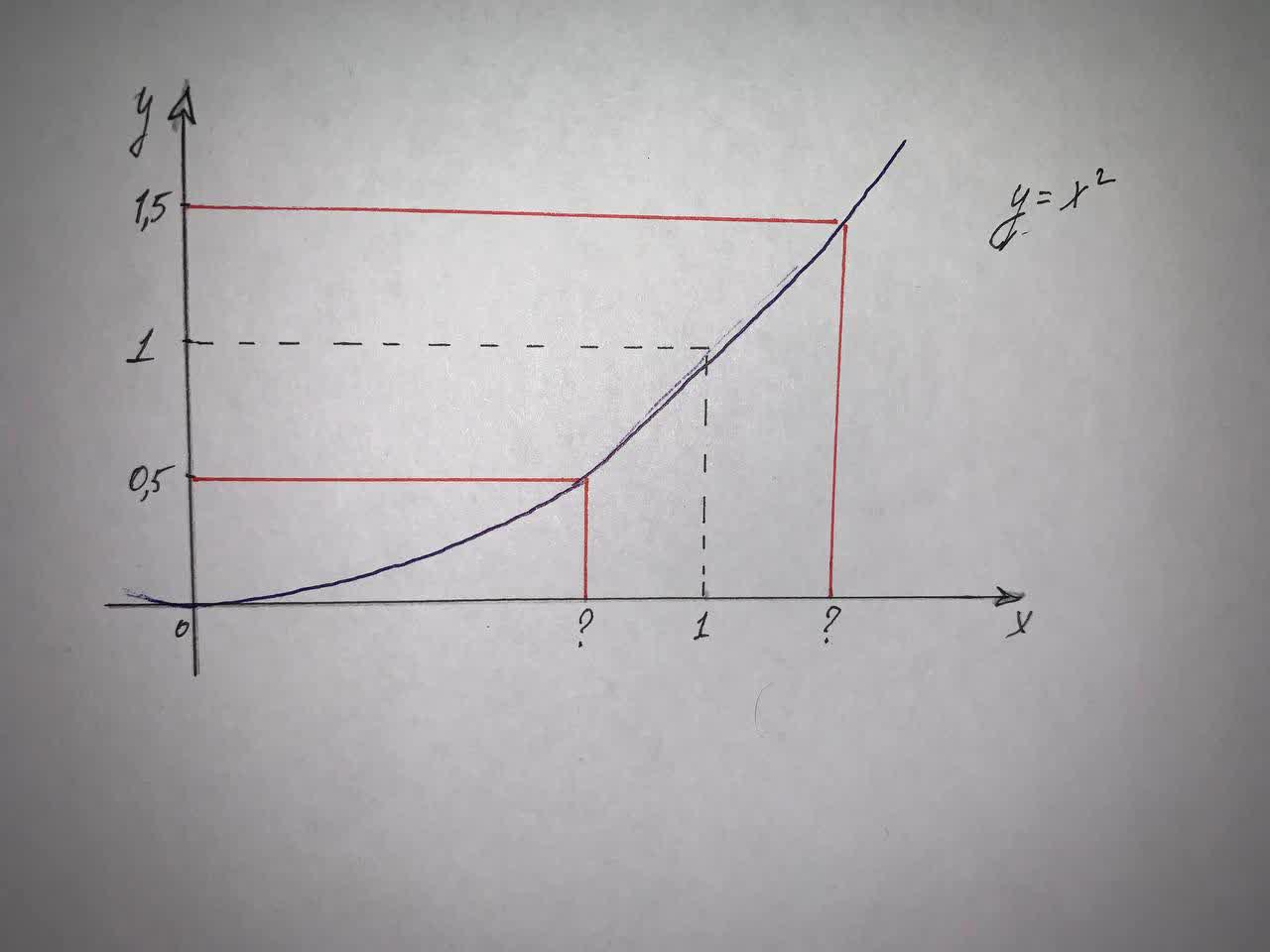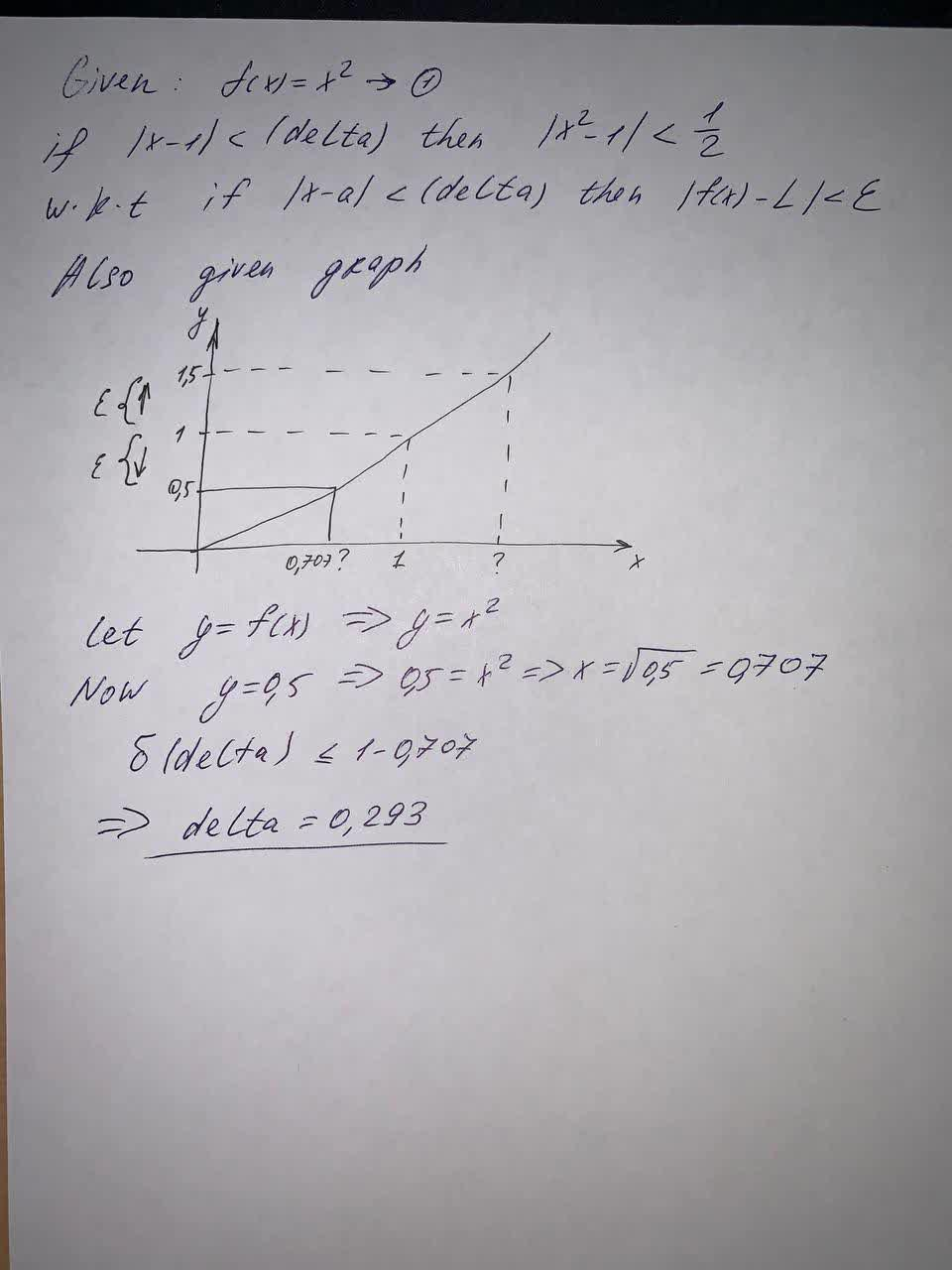Use the given graph off(x) = x^2to find a numberruigE 2021-08-14 Answered

Use the given graph of
$$\displaystyle{f{{\left({x}\right)}}}={x}^{{2}}$$
to find a number (delta) such that
if |x - 1| < (delta) then $$\displaystyle{\left|{x}^{{2}}-{1}\right|}{<}{\frac{{{1}}}{{{2}}}}$$
(delta) =• Questions are typically answered in as fast as 30 minutes

Solve your problem for the price of one coffee

• Math expert for every subject
• Pay only if we can solve itopsadnojD
A number (delta) will be